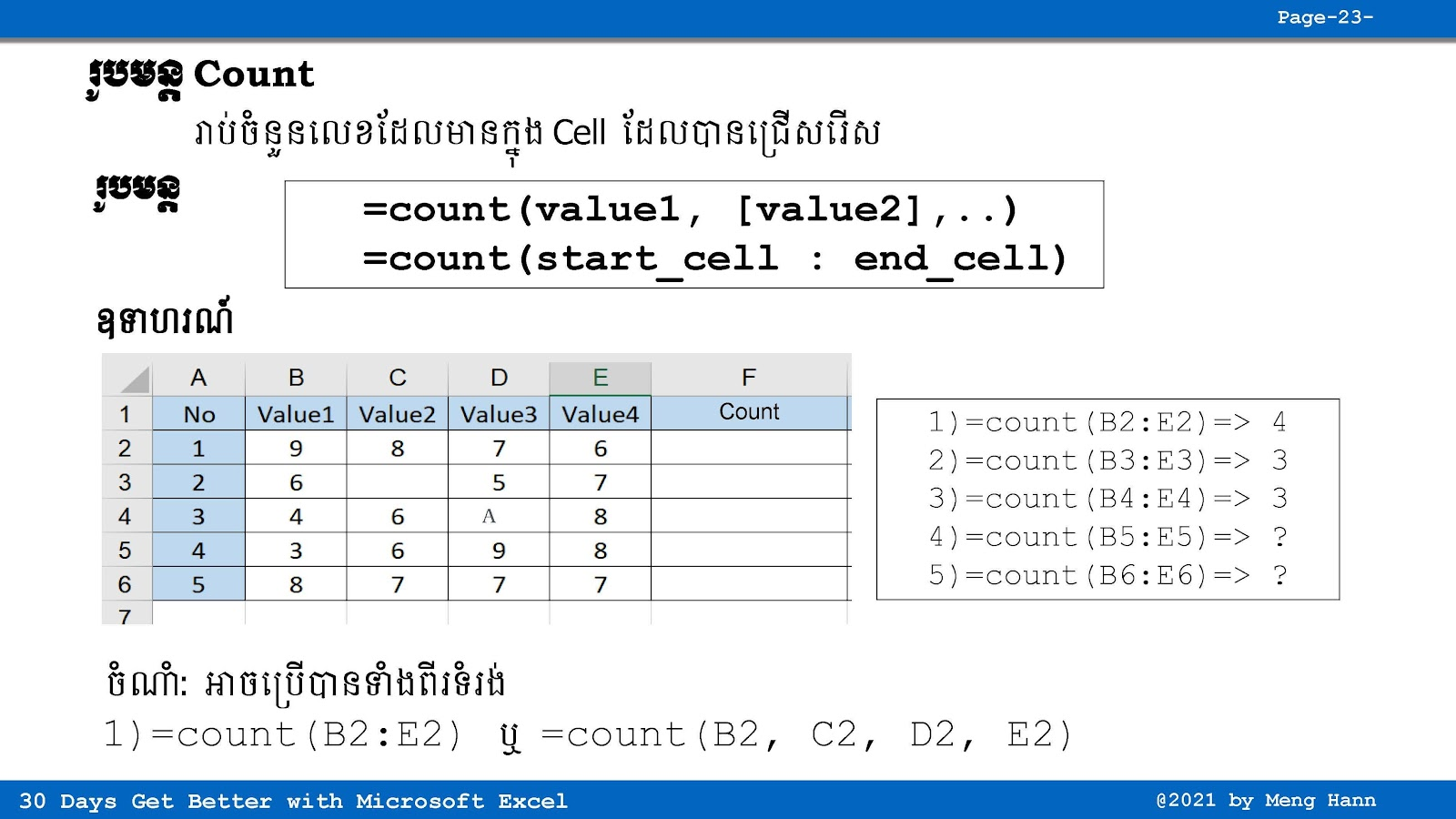# 21. How to use Count Formula in Excel speak Khmer

21. How to use Count Formula in Excel speak Khmer
This Excel tutorial in Khmer show how to use Count Formula to count only value from range of cells. Note: Text or other value not count, count only number value. Count Formula: Count only number as value of selected cells
=Count(Number1,[Number2],...)
=Count(start_cell:end_cell)
◾ Number1: Required, value to count, (number1,...,number255)
◾ start_cell: Required, start value to count.
◾ end_cell: Required, end value to count.